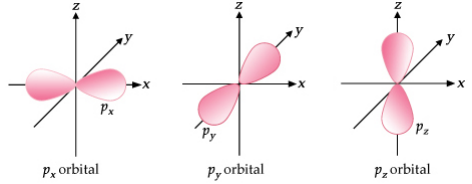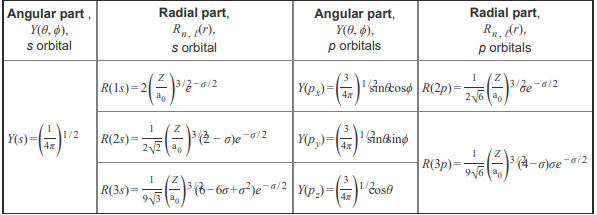# Problem: Part B. Each principal level with n = 2 or greater contains three p orbitals (px , py , and pz). The three 2p orbitals are shown below. The p orbitals are not spherically symmetric like the s orbitals, but rather have two lobes of electron density on either side of the nucleus. The three 2p orbitals differ only in their orientation, and they are perpendicular to one another.The 2p orbital is not spherically symmetric because Check all that apply.a) there are angular nodes in the yz, xz, and xy planes. b) the value of ψ 2 is zero at the nucleus. c) the 2p orbital has no angular nodes. d) the angular wave function of the 2p orbital is a constant. e) the angular wave function of the 2p orbital depends on the values of θ and ϕThe atomic orbitals are wave functions (ψ) that are mathematical solutions of the Schrödinger wave equation, and the atomic orbitals are represented mathematically by ψ(r, θ, ϕ) = R(r)Y(θ, ϕ)The function R(r) is called the radial wave function, where r is the distance of the electron from the nucleus of an atom where the probability of finding an electron is at a maximum, and the function Y(θ, ϕ) is called the angular wave function, where θ and ϕ represent different angles describing the orientation of the distance line, r , with respect to the x, y, and z axes. The electron probability density is expressed in terms of ψ2 . It is the probability (per unit volume) of finding the electron at a point in space. The region where electron probability density is zero is called a node. A node may be an angular node or a radial node. The solutions to the Schrödinger wave equation are characterized by three quantum numbers: n,ℓ, and mℓ . 1. The principal quantum number n determines the size and energy of the orbital. 2. The orbital angular momentum (or azimuthal) quantum number ℓ determines the angular distribution or the shape of an orbital. 3. The magnetic quantum number mℓ determines the orientation of the orbital. Angular and radial wave functions for s and p orbitalsThe equations for the angular and radial wave functions for the s and p orbital may be useful when completing this tutorial.

###### FREE Expert Solution
90% (166 ratings)View Complete Written Solution
###### Problem Details

Part B. Each principal level with n = 2 or greater contains three p orbitals (px , py , and pz). The three 2p orbitals are shown below. The p orbitals are not spherically symmetric like the s orbitals, but rather have two lobes of electron density on either side of the nucleus. The three 2p orbitals differ only in their orientation, and they are perpendicular to one another.The 2p orbital is not spherically symmetric because

Check all that apply.

a) there are angular nodes in the yz, xz, and xy planes.

b) the value of ψ 2 is zero at the nucleus.

c) the 2p orbital has no angular nodes.

d) the angular wave function of the 2p orbital is a constant.

e) the angular wave function of the 2p orbital depends on the values of θ and ϕ

The atomic orbitals are wave functions (ψ) that are mathematical solutions of the Schrödinger wave equation, and the atomic orbitals are represented mathematically by

ψ(r, θ, ϕ) = R(r)Y(θ, ϕ)

The function R(r) is called the radial wave function, where r is the distance of the electron from the nucleus of an atom where the probability of finding an electron is at a maximum, and the function Y(θ, ϕ) is called the angular wave function, where θ and ϕ represent different angles describing the orientation of the distance line, r , with respect to the x, y, and z axes.

The electron probability density is expressed in terms of ψ2 . It is the probability (per unit volume) of finding the electron at a point in space. The region where electron probability density is zero is called a node. A node may be an angular node or a radial node.

The solutions to the Schrödinger wave equation are characterized by three quantum numbers: n,, and m

1. The principal quantum number n determines the size and energy of the orbital.

2. The orbital angular momentum (or azimuthal) quantum number ℓ determines the angular distribution or the shape of an orbital.

3. The magnetic quantum number mℓ determines the orientation of the orbital.

Angular and radial wave functions for s and p orbitals

The equations for the angular and radial wave functions for the s and p orbital may be useful when completing this tutorial.